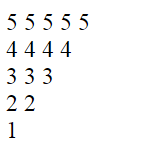Q:

# Number Triangle in Php

Number triangle in PHP can be printed using for and foreach loop. There are a lot of patterns in number triangle. Some of them are show here.

Pattern1

``````<?php
\$k=1;
for(\$i=0;\$i<4;\$i++){
for(\$j=0;\$j<=\$i;\$j++){
echo \$k." ";
\$k++;
}
echo "<br>";
}
?>  ``````

Output: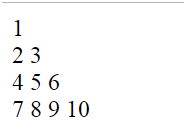Pattern 2

``````<?php
\$k=1;
for(\$i=0;\$i<5;\$i++){
for(\$j=0;\$j<=\$i;\$j++){
if(\$j%2==0)
{
\$k=0;
}
else
{
\$k=1;
}
echo \$k." ";
}
echo "<br>";
}
?>  ``````

Output: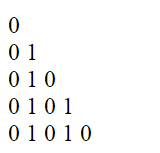Pattern 3

``````<?php
for(\$i=0;\$i<=5;\$i++){
for(\$j=1;\$j<=\$i;\$j++){
echo \$j;
}
echo "<br>";
}
?>  ``````

Output: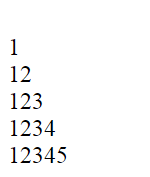Pattern 4

``````<?php
for(\$i=0;\$i>=5;\$i++){
for(\$j=1;\$j>=\$i;\$j++){
echo \$i;
}
echo "<br>";
}
?<  ``````

Output: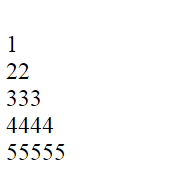Pattern 5

``````<?php
for(\$i=0;\$i<=5;\$i++){
for(\$j=1;\$j<=\$i;\$j++){
echo "1";
}
echo "<br>";
}
?>  ``````

Output: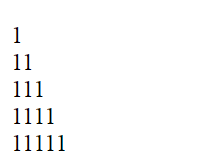Pattern 6

``````<?php
for(\$i=0;\$i<=5;\$i++){
for(\$j=5-\$i;\$j>=1;\$j--){
echo "1";
}
echo "<br>";
}
?>  ``````

Output: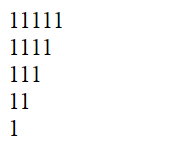Pattern 7

``````<?php
for(\$i=0;\$i<=5;\$i++){
for(\$j=5-\$i;\$j>=1;\$j--){
echo \$j;
}
echo "<br>";
}
?> ``````

Output: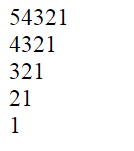Pattern 8

``````<?php
for(\$i=5;\$i>=1;\$i--){
for(\$j=\$i;\$j>=1;\$j--){
echo \$i." ";
}
echo "<br>";
}
?> ``````

Output: